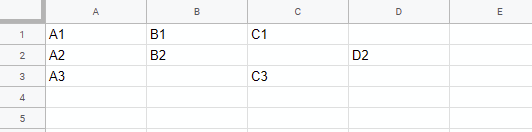# Sorting for Slice using Golang

Gists

This is a sample script for sorting a slice using Golang. Recently, I had a situation for sorting the coordinates of cells of Spreadsheet. As a test case, it thinks of the situation that the randomized cells are sorted. I think that this can be also used for a table except for Spreadsheet.

## Sample slice:

The sample slice is as follows.

ar := []struct {
row   int
col   int
value string
}{
{0, 0, "A1"},
{0, 1, "B1"},
{0, 2, "C1"},
{1, 0, "A2"},
{1, 1, "B2"},
{1, 3, "D2"},
{2, 0, "A3"},
{2, 2, "C3"},
}


When each element of above slice is put to a Spreadsheet, it becomes as follows.At the following sample script, after above element is randomized, the original slice is retrieved.

## Sample script 1:

Go Playground

package main

import (
"fmt"
"math/rand"
"sort"
)

func main() {
// Original slice
ar := []struct {
row   int
col   int
value string
}{
{0, 0, "A1"},
{0, 1, "B1"},
{0, 2, "C1"},
{1, 0, "A2"},
{1, 1, "B2"},
{1, 3, "D2"},
{2, 0, "A3"},
{2, 2, "C3"},
}

fmt.Println(ar)

// Randomize slice
// https://stackoverflow.com/a/12267471
for i := range ar {
j := rand.Intn(i + 1)
ar[i], ar[j] = ar[j], ar[i]
}

fmt.Println(ar)

// Sort
sort.Slice(ar, func(i, j int) bool {
if ar[i].row < ar[j].row {
return true
} else if (ar[i].col < ar[j].col) && (ar[i].row == ar[j].row) {
return true
}
return false
})

fmt.Println(ar)
}


### Result:

[{0 0 A1} {0 1 B1} {0 2 C1} {1 0 A2} {1 1 B2} {1 3 D2} {2 0 A3} {2 2 C3}]
[{1 3 D2} {1 1 B2} {0 2 C1} {2 0 A3} {2 2 C3} {0 0 A1} {1 0 A2} {0 1 B1}]
[{0 0 A1} {0 1 B1} {0 2 C1} {1 0 A2} {1 1 B2} {1 3 D2} {2 0 A3} {2 2 C3}]


## Sample script 2:

When row and col are switched as follows, the transposed result is obtained.

Go Playground

package main

import (
"fmt"
"math/rand"
"sort"
)

func main() {
// Original slice
ar := []struct {
row   int
col   int
value string
}{
{0, 0, "A1"},
{0, 1, "B1"},
{0, 2, "C1"},
{1, 0, "A2"},
{1, 1, "B2"},
{1, 3, "D2"},
{2, 0, "A3"},
{2, 2, "C3"},
}

fmt.Println(ar)

// Randomize slice
// https://stackoverflow.com/a/12267471
for i := range ar {
j := rand.Intn(i + 1)
ar[i], ar[j] = ar[j], ar[i]
}

fmt.Println(ar)

// Sort
sort.Slice(ar, func(i, j int) bool {
if ar[i].col < ar[j].col {
return true
} else if (ar[i].row < ar[j].row) && (ar[i].col == ar[j].col) {
return true
}
return false
})

fmt.Println(ar)
}


### Result:

[{0 0 A1} {0 1 B1} {0 2 C1} {1 0 A2} {1 1 B2} {1 3 D2} {2 0 A3} {2 2 C3}]
[{1 3 D2} {1 1 B2} {0 2 C1} {2 0 A3} {2 2 C3} {0 0 A1} {1 0 A2} {0 1 B1}]
[{0 0 A1} {1 0 A2} {2 0 A3} {0 1 B1} {1 1 B2} {0 2 C1} {2 2 C3} {1 3 D2}]